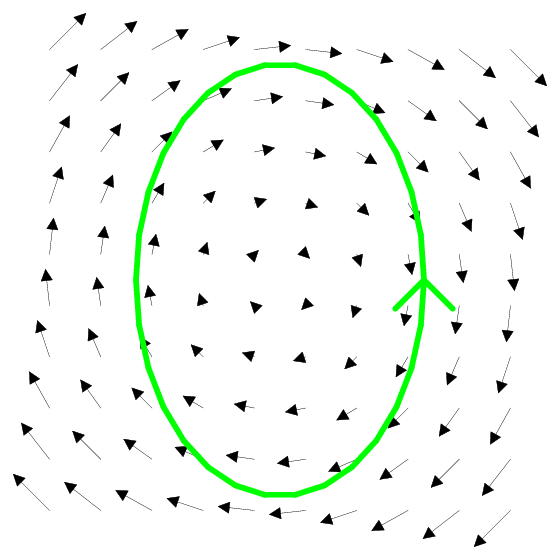# Math Insight

### Image: A 2D circulating vector field with closed curveThe vector field $\dlvf(x,y) = (y,-x)$ tends to circulate in the clockwise direction. The green curve is the ellipse \begin{align*} \frac{x^2}{4}+\frac{y^2}{9} =1 \end{align*} oriented counterclockwise. The circulation calculated by the line integral of $\dlvf$ over the curve should be negative.

Image file: vector_field_circulate_closed_curve.png